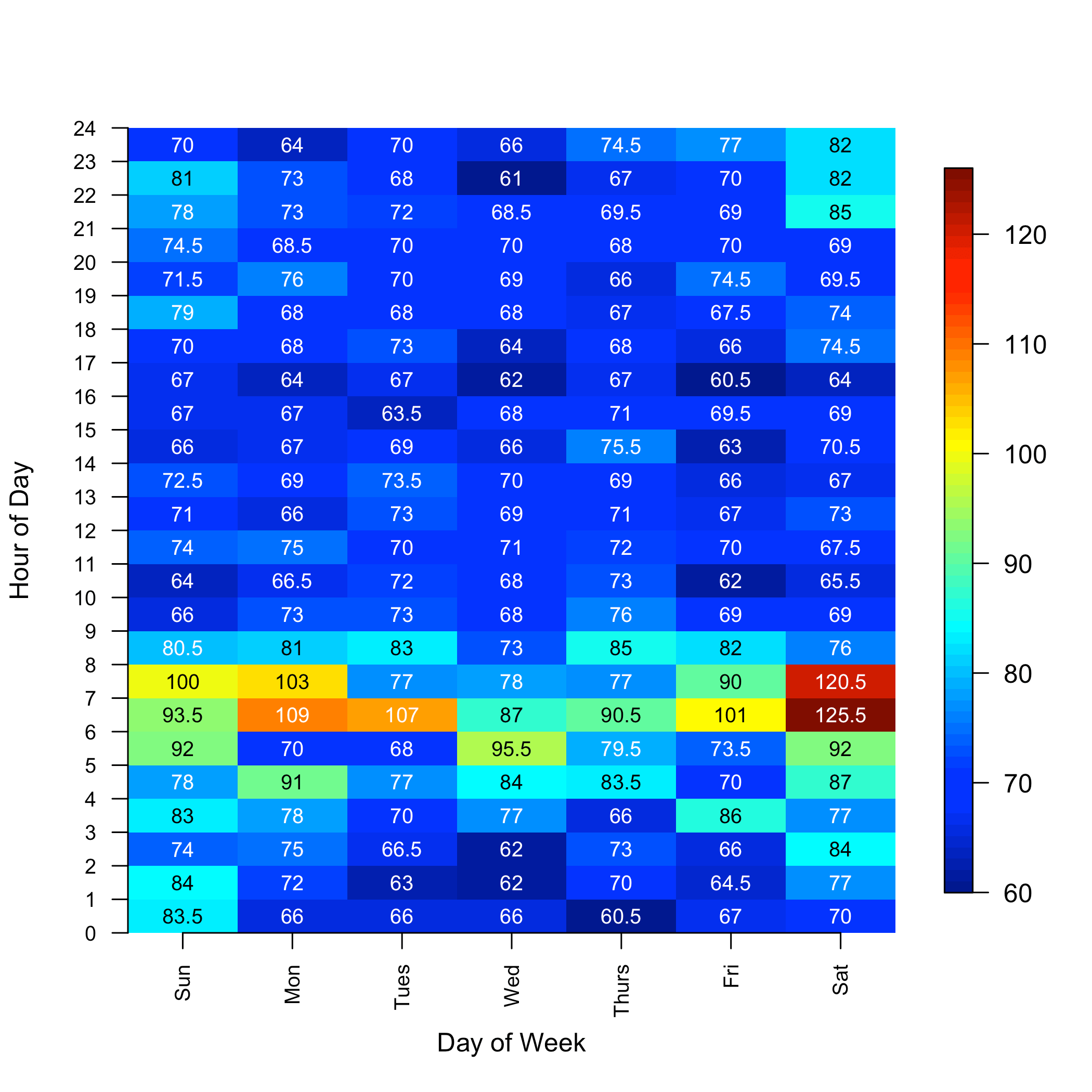Want to share your content on R-bloggers? click here if you have a blog, or here if you don't.

## The Problem

In two previous posts, I discussed visualizing your turnaround times (TATs). These posts are here and here. One other nice way to visualize your TAT is by means of a heatmap. In particular, we would like to look at the TAT for every hour of the week in a single figure. This manner of dataviz bling seems to be particularly attractive to managers because it costs you \$0 to do this with R, but with commercial tools like Tableau, you'd have to pay a fortune and, as with Excel, your report would not be readily reproducible. Further, to make it autogenerate a PDF would mean you had to fork out more money for a report-generation module. Pffft.

## The Data

We're going to read in a year's worth of order times and result times for a stat immunoassay test offered to a particular ward. The data, as I've formatted it, has two columns, ord and res.

```test.data <- read.csv("test_data.csv")

```##                   ord                 res
## 1 2015-01-01 13:24:00 2015-01-01 14:29:00
## 2 2015-01-01 06:16:00 2015-01-01 07:43:00
## 3 2015-01-01 06:32:00 2015-01-01 07:43:00
## 4 2015-01-01 06:32:00 2015-01-01 07:43:00
## 5 2015-01-01 12:12:00 2015-01-01 13:13:00
## 6 2015-01-01 12:12:00 2015-01-01 13:13:00```

Now, of course, we want to look at data collected from a long period of time so that we can be sure that the observations we are not simply an artifact of recent instrument downtime, maintenance, or whoever happened to be running the instrument. This is why I chose a year's worth of data. We are going to visualize the median order-to-file TAT for this test.

## Formatting and Calculations

To calculate the hourly medians, we'll need to be able to label every TAT with the day it was run and the hour in the day it was run. This is pretty easy with the lubridate package. We'll do three things:

• We'll convert the dates to POSIXct objects
• We'll use the `difftime()` function to calculate the TATs
• We'll use the `wday()` function to determine which day of the week the specimen was run on
• We'll pull out the hour of the day on which it was run with the `format()` function.

```library("dplyr")
library("lubridate")
library("fields")
library("magrittr")

test.data\$ord <- ymd_hms(test.data\$ord)
test.data\$res <- ymd_hms(test.data\$res)
test.data <- mutate(test.data,otf = difftime(res,ord,units="min"))
test.data <- mutate(test.data,dow = wday(ord))
test.data <- mutate(test.data,hod = as.numeric(format(test.data\$ord, "%H")))```

And now the data will look like this:

`head(test.data)`

```##                   ord                 res     otf dow hod
## 1 2015-01-01 13:24:00 2015-01-01 14:29:00 65 mins   5  13
## 2 2015-01-01 06:16:00 2015-01-01 07:43:00 87 mins   5   6
## 3 2015-01-01 06:32:00 2015-01-01 07:43:00 71 mins   5   6
## 4 2015-01-01 06:32:00 2015-01-01 07:43:00 71 mins   5   6
## 5 2015-01-01 12:12:00 2015-01-01 13:13:00 61 mins   5  12
## 6 2015-01-01 12:12:00 2015-01-01 13:13:00 61 mins   5  12```

where the order-to-file TAT is in the otf column, the day-of-week is in the dow column and the hour-of-day is in the hod column. Now we can cycle though the days of the week and the hours of the day and calculate the year's median TAT for each hour, storing it in a matrix:

```#prepare an empty matrix
heat.data <- matrix(rep(NA,7*24),nrow = 7, ncol = 24)
#loop over the days and hours and calculate the median TAT
for(i in 1:7){
for(j in 0:23){
heat.data[i,j+1] <- subset(test.data, test.data\$dow==i & test.data\$hod==j)\$otf %>% median
}
}```

## Making the Heatmap

There are many ways to make the heatmap but I am particularly fond of the appearance of surface plots made with the fields package.

```image.plot(1:7,seq(from=0.5, to=23.5, by = 1),heat.data,axes=FALSE,
xlab = "Day of Week", ylab = "Hour of Day", ylim=c(0,24))
# the following pointless command is necessary to make the custom axis labels non-transparent
# google revealed this among a number of other workarounds.
points(0,0)
# now these will display properly
axis(side=1, at=1:7, labels=as.character(wday(1:7, label=TRUE)), las=2, cex.axis = 0.8)
axis(side=2, at= 0:24, labels=0:24, las=1, cex.axis=0.8)```## Overlay Printed Times

We can see that there is a morning slowdown that is particularly bad on Saturday. But what if we wanted to know the exact value for these eye-catching problem times? We'd have trouble, unless we overlaid some text.

It turns out that if you use white printing, you can't read the numbers when the background colour is yellow and green. There is a 64 colour gradient used in the `image.plot()` function, so I calculated which integers in 0–64 were the problem and found the TATs that would correspond. It turned out that colours 20–45 out of the 64 colours in the gradient are the problem. By this means, I can make the printing black over the yellows and greens but white everywhere else:

```image.plot(1:7,seq(from=0.5, to=23.5, by = 1),heat.data,axes=FALSE,
xlab = "Day of Week", ylab = "Hour of Day", ylim=c(0,24))
points(0,0) #random command that resets par
axis(side=1, at=1:7, labels=as.character(wday(1:7, label=TRUE)), las=2, cex.axis = 0.8)
axis(side=2, at= 0:24, labels=0:24, las=1, cex.axis=0.8)

# calculate the lowest and highest TAT
min.z <- min(heat.data)
max.z <- max(heat.data)
# determine which TAT's will have yellow to green shading
z.yellows <- min.z + (max.z - min.z)/64*c(20,45)
# print the labels
for(i in 1:7){
for(j in 1:24){
if((heat.data[i,j] > z.yellows)&(heat.data[i,j] < z.yellows)){
text(i,j-0.5,heat.data[i,j], col="black", cex = 0.8)
}else{
text(i,j-0.5,heat.data[i,j], col="white", cex = 0.8)
}
}
}```So, that is not too bad, and if you wanted to look at the 75th percentile instead you would only have to adjust the heat.data calculation as follows:

```#prepare an empty matrix
heat.data <- matrix(rep(NA,7*24),nrow = 7, ncol = 24)
#loop over the days and hours and calculate the median TAT
for(i in 1:7){
for(j in 0:23){
heat.data[i,j+1] <- subset(test.data, test.data\$dow==i & test.data\$hod==j)\$otf %>% quantile(.,probs=0.75)
}
}```

And this is what you will get.Hmmm…we'd better look at Saturday morning, 6 am. I hope you have found this helpful.

And as for heat

“He will sit as a refiner and purifier of silver”

Malachi 3:3